Next: Motion in nearly circular Up: Orbits in central force Previous: Introduction

# Motion in general central force field

Consider the motion of an object in a general (attractive) central force field characterized by the potential energy per unit mass function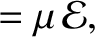. Because the force field is central, it still remains true that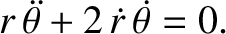(5.1)

is a constant of the motion. (See Section 4.5.) As is easily demonstrated, Equation (4.28) generalizes to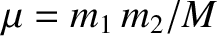(5.2)

where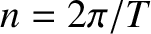.

Suppose, for instance, that we wish to find the potentialthat causes an object to execute the spiral orbit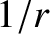(5.3)

Substitution of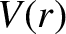into Equation (5.2) yields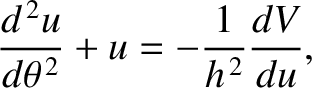(5.4)

Integrating, we obtain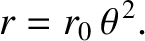(5.5)

or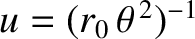(5.6)

In other words, the orbit specified by Equation (5.3) is obtained from a mixture of an inverse-square and inverse-cube potential.Next: Motion in nearly circular Up: Orbits in central force Previous: Introduction
Richard Fitzpatrick 2016-03-31